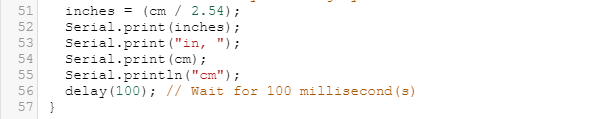Search

# Measuring Distance With Sound

Updated: Jun 7, 2020

Have you ever measured distances? Of course you did, but have you done it with the help of sound? Yes… we can calculate the distance of an object with the help of sound which is easy to make and very exciting. Tinkercad gives you an amazing platform where you can make this project and run simulations. Let’s check it out.

THE CIRCUIT-In the search bar you have to search “ultrasonic range finder” and you will find a prebuilt circuit which you have to put it in the open space.

The circuit consists of an “Arduino Uno” and a “Ping Ultrasonic Range Finder”. The ground (GND) terminal of the “Ping” is connected to the ground pin of the Arduino. Then we are supply 5V voltage to the “Ping” by connecting the voltage terminal of the “Ping” to the 5V pin of the Arduino. At last we connect the signal (SIG) terminal of the “Ping” to the 7th pin of the Arduino.

WORKING-

The signal is sent by the “Ping” which after hitting the object gets reflected back at the “Ping” then that information is sent to the Arduino which then calculates how long did it take for the signal to reach the “Ping” and then it calculates the distance of the object from the “Ping”.

CODE-

The code is prewritten for the circuit which makes it easier to run. The explanation of the code is down below.Line 22 and 24 are initializing the variables “inches” and “cm” as 0.

Line 26: long readUltrasonicDistance(int triggerPin, int echoPin)

This is the function in which the Ping is commanded how to send signals and the values of the “triggerPin” and “echoPin” will be sent to this function which then will be processed.

Line 28-30: pinMode(triggerPin, OUTPUT); // Clear the trigger

digitalWrite(triggerPin, LOW);

delayMicroseconds(2);

The “triggerPin” is set as output and low voltage is applied i.e 0. This will send a null signal (no signal) which is important to send a clear high voltage signal on the next round.

Line 32-35: digitalWrite(triggerPin, HIGH);

delayMicroseconds(10);

digitalWrite(triggerPin, LOW);

pinMode(echoPin, INPUT);

Next the “triggerPin” is set to “HIGH” which will make the “Ping” to send signal . After that we can see that the “triggerPin” is again set to “LOW” which is important so that the “Ping” is at initial condition again.

Line 40-44: void setup()

{ Serial.begin(9600); }

This is the setup() function where the serial connection of the Arduino is initialized at 9600 bits. 9600 bits per second is the default for the Arduino, and is perfectly adequate for the majority of users, but you could change it to other speeds.

Line 46-57: void loop()

{

// measure the ping time in cm

cm = 0.01723 * readUltrasonicDistance(7, 7);

// convert to inches by dividing by 2.54

inches = (cm / 2.54);

Serial.print(inches);

Serial.print("in, ");

Serial.print(cm);

Serial.println("cm");

delay(100); // Wait for 100 millisecond(s)

}

This loop() function calculates the time taken by the signal to come back after hitting the object and calculates the distance in cm and inches. It also prints the distances of all the objects that the signal has measured.

OUTPUT-After running the simulation you will notice a blue circle (object ) which you can drag around to get different measurements of the distance.

Like this you can calculate the distance of objects with style.

NOTE- The range of the “Ping” is from 3cm to 400cm.

Written by

Shaswat Baruah